# Convert Minutes to Hours in Excel & Google Sheets

This tutorial will demonstrate how to convert minutes to hours or hours to minutes in Excel & Google Sheets.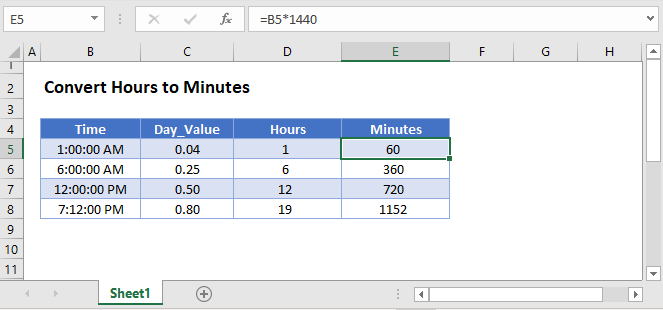## Convert Hours to Minutes

To convert hours to minutes all you need to do is multiply the hours by 60: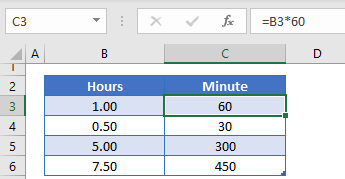Or you could use the CONVERT Function:

``=CONVERT(B3,"hr","mn")``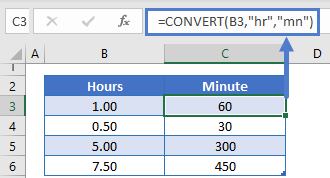## Convert Minutes to Hours

To convert minutes to hours all you need to do is divide the minutes by 60: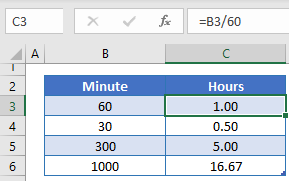Or you could use the CONVERT Function:

``=CONVERT(B4,"mn","hr")``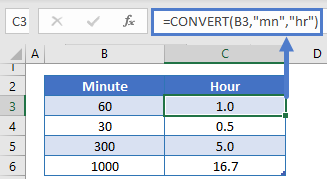## Convert Time to Minutes or Hours

### Convert Time to Hours

Excel stores time as a decimal value where each 1/24th represents one hour of a day. Understanding this, you can convert a time to hours by multiplying the time by 24: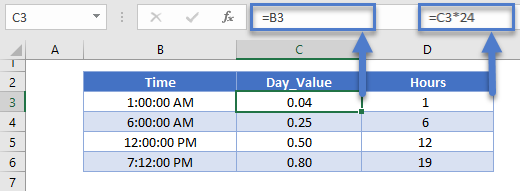### Convert Time to Minutes

You can convert time to minutes by multiplying the result by an additional 60 (Time * 24 * 60 = number of minutes):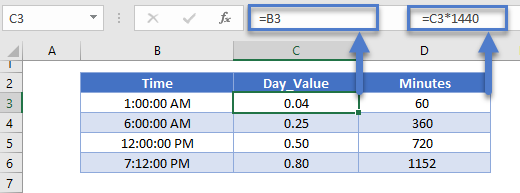### Format as Number

When you convert time to hours or minutes, Excel may automatically format the result as time (ex. h:mm):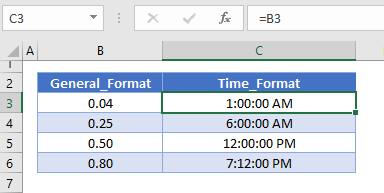To display the result as a regular number, change the formatting to General: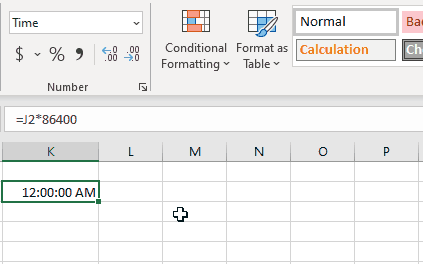## Convert Minutes to Hours in Google Sheets

All of the above examples work exactly the same in Google Sheets as in Excel.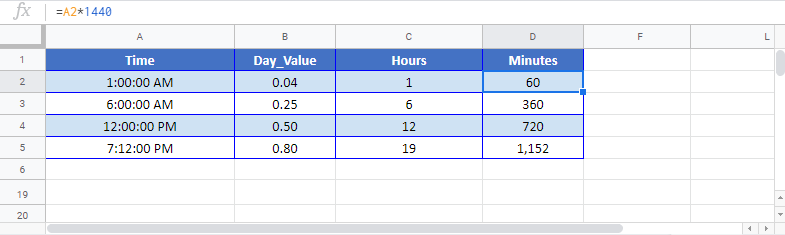### Excel Practice Worksheet

Practice Excel functions and formulas with our 100% free practice worksheets!